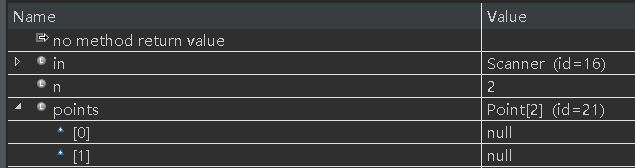The source code is as follows:

```        Point[] points = new Point[n];//Point is a class
for ( int i=0;i<n;i++ ) {
points[i]. setX(in.nextInt());//The 29th line
points[i].setY(in.nextInt());
}```

The error message is as follows:

at test.Main.zjd(Main.java:29)
at test.Main.main(Main.java:9)

analysis:

```Point[] points=new Point[n];//After defining the class array, I thought that the above code could be used directly, but the above error was reported.
So I set line 29 as a breakpoint, and
when I started Debug and run to this point, I found the problem:```

It is found in the variable list that the values ​​of points and points are all null

It seems that there is no problem at a glance, but when you think about it, each element in the array is an object. Since it is an object, how can we use it without allocating space (without new)?

Modified code:

```        Point[] points= new Point[n];
for ( int i=0;i<n;i++ ) {
points[i] =new Point(); //Solution: apply for allocating space for the elements in the class array here .